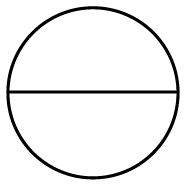### Home > ACC7 > Chapter 9 Unit 6 > Lesson CC2: 9.1.2 > Problem9-31

9-31.The circle shown at right has a diameter of $20$ cm.

1. What measurement do you need to find in order to calculate the area inside the circle?

Recall the equation for area from the Math Notes box in this lesson.
$A=r^2\pi$

What measurement is missing in this equation?

2. Find the area inside the circle using the formula $A=r^2\pi$. Write your answer as a product of $r^2$ and $\pi$, and as an approximation using $\pi=3.14$.

Solve for $r$.
$20=2r$
$A=(10)^2\pi$
$314$ cm2